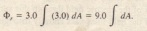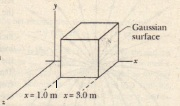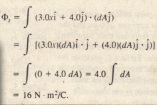# Sample Problem

Sample Problem

A nonuniform electric  field given by pierces the Gaussian cube shown is in newtons per coulomb and x is in meters.) What is  electric flux through the right face the left face and the top face The Key Idea here is that we can find the flux <I> through the surface by integrating the scalar product g over each face Right face An area vector A is always perpendicular to its surface and always points away from the interior of a Gaussian surface Thus the vectorfor the right face of the cube must point in the positive  direction unit vector notation then From the flux through the right face is then.We are about to integrate over the right face but we note that  has the same value everywhere on that face-s-namely  This means we can substitute that constant value for Then.Now the integral merely gives us the area A = 4.0 m of the right face so<l>r = (9.0 N/C)(4.0 m~) = 36 N . m/C. (Answer) Gaussian cube with one edge on the x axis lies within a nonuniform electric field.Left face The procedure for finding the flux through the left face is the same as that for the right face However two factors change The differential area vector points in the negative  direction and thus The term  again appears in
our integration and it is again constant over the face being considered However on the left face With these two changes we find that the flux through the left face is Top face The differential area vector points in the positive direction and thus The flux through the top face.